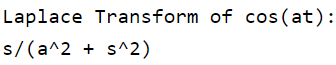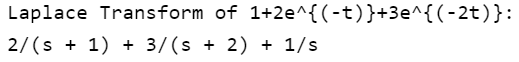Related Articles

# Laplace Transform in MATLAB

• Difficulty Level : Easy
• Last Updated : 21 Apr, 2021

In this article, we will see how to find Laplace Transform in MATLAB. Laplace Transform helps to simplify problems that involve Differential Equations into algebraic equations. As the name suggests, it transforms the time-domain function f(t) into Laplace domain function F(s).Using the above function one can generate a Laplace Transform of any expression.

Example 1: Find the Laplace Transform of.

## Matlab

 % specify the variable a, t and s as symbolic ones % The syms function creates a variable dynamically % and automatically assigns to a MATLAB variable% with the same namesyms a t s  % define function f(t) f=cos(a*t);  % laplace command to transform into % Laplace domain function F(s)F=laplace(f,t,s);    % Display the output valuedisp('Laplace Transform of cos(at):')disp(F);

Output:Example 2: Find the Laplace Transform of a particular expression i.e. 1+2e^{(-t)}+3e^{(-2t)}.

## Matlab

 % specify the variable a, t and s % as symbolic ones  syms a t s e  % define function f(t) f=1+2*exp(-t)+3*exp(-2*t);  % laplace command to transform into % Laplace domain function F(s)F=laplace(f,t,s);    % Display the output valuedisp('Laplace Transform of 1+2e^{(-t)}+3e^{(-2t)}:')disp(F);

Output:My Personal Notes arrow_drop_up